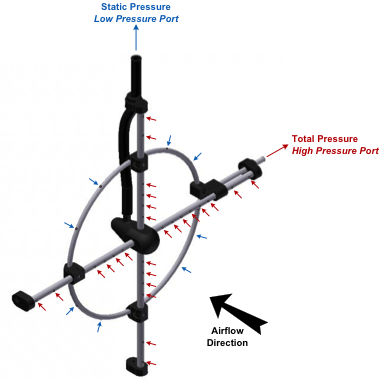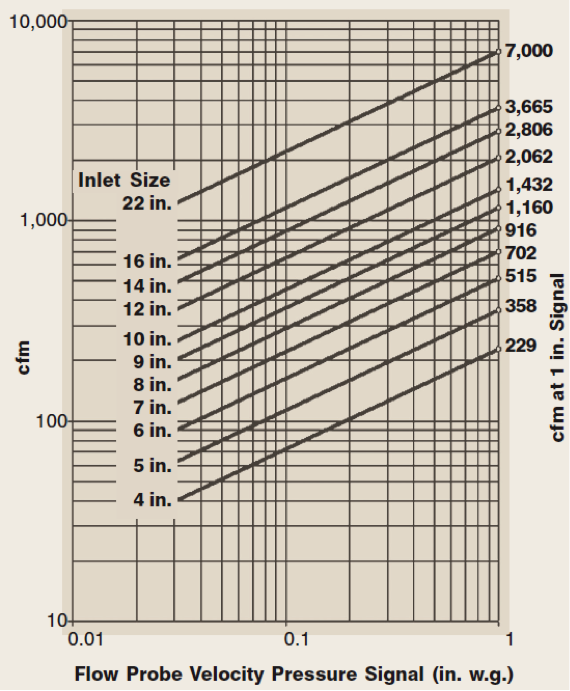Home TechTime Controls The K factorThe K factor

Perfecting airflow

When dealing with pressure independent VAV boxes, we are often asked if the K factor is important, what it is and where this information can be found. The answer to the first question is a definite “yes", the K factor is important. The other two questions are a little more complex and will require a bit of explanation.

The K factor is Neptronic’s solution to what is otherwise a complicated process in configuring your VAV box for optimal airflow reading. For clarity, let’s start with measuring the airflow. This is achieved with the help of crossflows. It can also be done with pitot tubes, but the reading would not be as accurate since it can only measure a single point in the duct work. The crossflow however, can take multiple point readings to average the entire duct work. Crossflow design varies among manufacturers but the principal remains the same. Below is an example of a cross flowAs you can see from the above crossflow, there are two pressure types that can be measured with this device: Total Pressure & Static Pressure. Total pressure can be measured using the holes facing the airflow (red arrows) and Static Pressure is measured using the holes located on the side of the ring (blue arrows). As shown on the drawing, total pressure is connected to the high pressure port and the static pressure is connected to the low pressure port.

Converting Velocity Pressure into Airflow

Inside the EVCB series controller, the integrated differential pressure transducer calculates the variable known as Velocity Pressure (also referred to as Differential Pressure ) based on the following formula.

Total Pressure - Static Pressure = Velocity Pressure

The differential pressure transducer produces a millivolt signal based on the velocity pressure reading. Based on the velocity pressure value, we can then transfer this information into an airflow reading.

The general formula to convert velocity pressure into airflow is the following;Most other manufacturers will require the size of the in order to calculate the area and use a correction factor of some sort (the Amplification Factor) in order to apply this general formula.

Amplification Factor

To find the amplification factor (which is differs among manufacturers), the following formula is applied;

F = (4005 * Area/Max Airflow)2

Airflow in this case is in CFM at 1" w.g. velocity pressure (given by VAV box manufacturers)

In order to avoid unnecessary calculations and simplify the process, Neptronic decided to merge some of the variables together (the K factor). As such, the following formula is used;

K Factor = Area * 4005 * √(Velocity Pressure @ 1" w.g./Amplification Factor)

For clarity, the K factor encompasses the Constant (4005 FPM @ 1" w.g), the Area (ft2), the Velocity Pressure (1" w.g.) and the Amplification Factor.

To find the K factor, simply refer to the information found in either a graph or chart format in the specifications of the VAV box (the chart includes the calculations of the area and Amplification factor). The K factor is the point at which the size of the box, shown in inches, converges with the maximum airflow at 1"w.g. velocity pressure (on the far right). See examples below.Based on the graph, we can see that the maximum airflow for a 6" VAV box is 515CFM. When configuring the EVCB controller K factor, 515 would be entered.

Based on the table (from another manufacturer), the same 6" VAV box will supply 435CFM. When configuring the EVCB controller K factor, 435 would be entered.

This is how you can find the K factor value to configure your EVCB series controller. The right K factor value will enable you to get more precise airflow reading out of your controller. The farther you are from the real K factor, the farther you will be from the right airflow reading and the harder it will be to balance the VAV box afterwards.